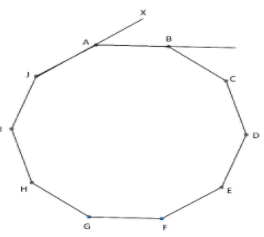QuestionAnswers

# The aperture of a camera is formed by 10 blades overlapping to from a regular decagon. What is the measure of $\angle BAX?$.A.${45^ \circ }$B.${36^ \circ }$C.${144^ \circ }$D.${44^ \circ }$

Hint: In this question we have given it is a decagon. So clearly it has 10 sides. So using the formula for the sum of interior angles of $n -$sided polygon we will get the sum of angles. After that using the formula for calculating each interior angle of a polygon $=$ $\dfrac{{\left( {n - 2} \right)180}}{n};$ where n is the number of sides of polygon.
We will get measure of each interior angle. Then using the formula: exterior angle $=$${180^ \circ } -$ interior angle we will get the required answer.We have given the aperture of a camera is formed by 10 blades overlap to form a regular decagon.
As we know the sum of interior angles of $n -$sided polygon $=$ $\left( {n - 2} \right) \times 180$.
Here, we have decagon
So, $n = 10$
Hence, sum of interior angles of decagon $= \left( {10 - 2} \right) \times {180^ \circ }$ $= 8 \times {180^ \circ }$
$\Rightarrow$Sum of angles of interior angles of decagon$= {1440^ \circ }$
Now, using the formula for calculating each interior angle of a polygon $=$ $\dfrac{{\left( {n - 2} \right)180}}{n};$ where n is the number of sides of polygon.
Here, $n = 10$ so we get,
Each interior angle of a decagon $=$ $\dfrac{{\left( {10 - 2} \right)180}}{{10}}$
$\Rightarrow \dfrac{{8 \times 180}}{{10}}$ $= 8 \times 18$
$\Rightarrow {144^ \circ }$
Hence, we get each interior angle of a decagon is of${144^ \circ }$.
So, from the figure we can say that $\angle JAB = {144^ \circ }$
Now,
Use the fact that, exterior angle $=$${180^ \circ } -$ interior angle
Here, we have$\angle JAB = {144^ \circ }$
Therefore, exterior angle of a decagon $= {180^ \circ } - {144^ \circ }$
$= {36^ \circ }$
From the figure we can say that $\angle BAX$is an exterior angle.
$\therefore \angle BAX = {36^ \circ }$
Thus, the measure of $\angle BAX$ is ${36^ \circ }$
Hence, option B. ${36^ \circ }$is the correct answer.

Note: Interior angle of a polygon is an angle inside a polygon at a vertex of the polygon.
Exterior angle of a polygon is an angle at a vertex of the polygon, outside the polygon, formed by one side and the extension of an adjacent side.
The sum of exterior angles of a polygon must be ${360^ \circ }$.